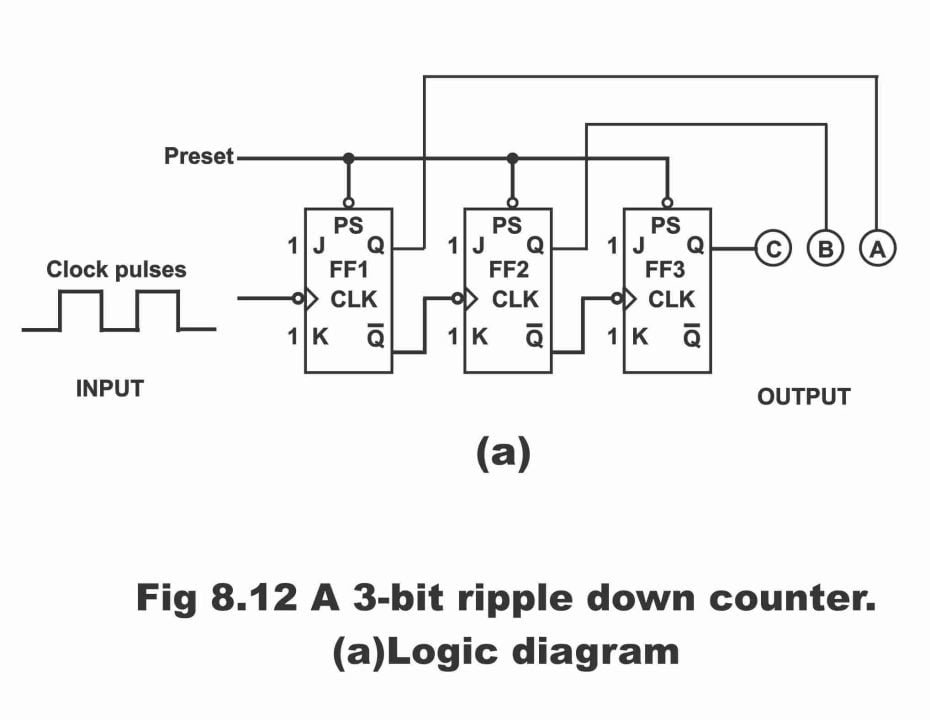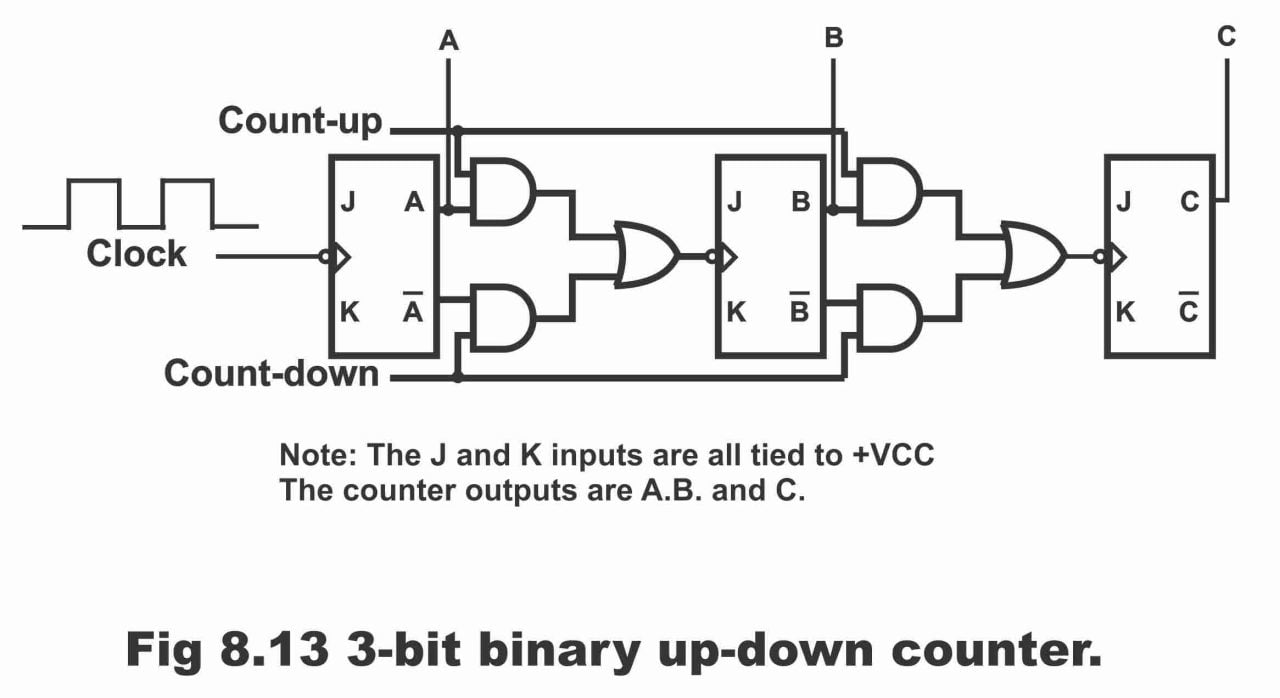Digital Electronics

# Down Counters and Up-Down Counters in Digital Electronics

## Down Counters

Until now, we have studied about counters which conduct counting in an upward direction (i.e. 0, 1, 2, 3, 4, 5 ….). These types of counters are called up-counters. In other words, counters, which count from a low number to a higher number, are called up-counters. Every time when a clock pulse strikes on an up-counter’s input, an addition of one digit occurs in the binary number existing inside the counter. In other words, as we have stated previously, as a result of consistently applying clock pulses on clock inputs of such a counter, the counter’s reading (i.e. binary numbers existing on the counter) increase. However, contrary to this, we can also count in downward direction in a digital system. As such, counters which count from a high binary number to a low number (i.e. 9, 8, 7, 6,5….) or counters which can count from high to low numbers, are called down counters. When a clock pulse is applied on clock input of a down counter, a shortfall of one digit occurs in maximum or high binary number existing within the counter. In other words, as a result of continuous clock pulses being received on down counters’ clock inputs, binary number present on it, keep tumbling continuously or a consistent decline in the counter’s reading occurs.

In figure 8.12 (a) a 3-bit ripple down counter or mod-8 asynchronous down counter (which counts from 000 to 111) has been illustrated. The counting sequence of this counter has been depicted in figure (b). If we cautiously go through the logic diagram (figure a), this particular counter just seems similar to an up-counter. The only exception being that instead of connecting normal output (i.e. Q) of every flip-flop in a down counter to the clock input of next flip-flop, complementary output (i.e. Q) of every flip-flop is connected to the clock input of next flip-flop. In other words, the basic difference between up-counter and down-counter is that of connecting one flip-flops ‘output to next flip-flop’s input. A normal output (i.e. Q) of every flip-flop is applied in the counter on the clock input of next flip-flop. Whereas in down counter, complement output of every flip-flop (i.e. Q) is connected with clock input of the next flip-flop. As such counting sequence in a down counter is totally reversed as compared to an up-counter, as has been exemplified in figure (b). It must be kept in mind that a preset control (which is denoted by PS or PRE) also exists in a down counter. Its job is to set counter on its maximum value (according to this figure decimal 7 or binary 111) and get- start downward count. Moreover, every flip-flop toggles (i.e. changes its state) only when the clock pulse being applied on its clock input turns from high to low (or from 1 to 0). (However, in an up counter, every flip -flop changes its state at a time only when its clock input turns from low to high). The operational mechanism of a down counter is as follows;

Figure 8.12-A 3-bit ripple down counter (a).Figure 8.12 (b) – counting sequence

 Number of Clock Pulses Binary Counting Sequence Decimal Count C B A 0 1 1 1 7 1 1 1 0 6 2 1 0 1 5 3 1 0 0 4 4 0 1 1 3 5 0 1 0 2 6 0 0 1 1 7 0 0 0 0 8 1 1 1 7 9 1 1 0 6

Firstly, this counter is pre- set on its high value. In such a situation, counters’ output will be as follows;

CBA = 111 (decimal 7)

When first clock pulse strikes, flip-flop FF1 (normal output of which is directly connected with output A) resets and its complement output Q, which is high, is received on flip-flop FF2 input. When the first flip-flop resets, counter’s output becomes as follows;

CBA = 110 (decimal 6)

On the striking of 2nd clock pulse, flip-flop FF1 which was previously reset, sets. However, flip-flop FF2 which was earlier set, resets. However, complement output Q, which is high, is received on the next flip-flop i.e. FF3. As a result of first flip-flop getting set and second’s flip-flop getting reset, counter’s output will be as follows;

CBA = 101 (decimal 5)

When the 3rd clock pulse strikes, only first flip-flop changes its state i.e. it resets. In such a situation, following output is obtained.

CBA = 100 (decimal 4)

When 4th clock pulse is applied, all three flip-flops change their conditions simultaneously. This has been illustrated in via a truth table as shown in figure (b), from which following output is received;

CBA = 011 (decimal 3)

On the 5th clock pulse, only first flip-flop toggles, as a result of which, it gets reset. Thus,

CBA = 010 (decimal 2)

On 6th clock pulse, first flip-flop sets whereas the second flip-flop resets, as has been depicted via figure (b). In such a situation, following output results;

CBA = 001 (decimal 1)

On the application of 7th clock pulse, only flip-flop FF1 toggles, as a result counters’ output becomes as under;

CBA = 000 (decimal 0)

On 8th clock pulse, all flip-flops return back simultaneously to their pre-set value (i.e. 111) and afore-mentioned process of down counting re-starts.  This has been illustrated in figure (b).

## Up-Down Counter

A basic counter (synchronous or asynchronous) which has capacity to count at a time either in upward or downward direction, is called up-down counter. In other words, if attributes of an up and down counters are combined together in a single counter, such a counter is called an up-down counter.

In figure 8.13, a 3-bit binary asynchronous up-down counter has been represented. In this counter, two AND gates and an OR gate are used in order to connect every flip-flop existing inside the counter with other. This has been explicated via figure. Normal output of every flip-flop is provided on one AND gate and complement output on other AND gate. Both these AND gates determine whether state of next flip-flop has to be changed through normal output or it has to be changed via complement output (because we know that if normal output of every flip-flop within a counter is received on every next flip-flop, then such a counter is up-counter. And if complement output of every flip-flop present inside the counter is received on every next flip-flop, such a counter is called down counter). Whether this up-down counter is required to be operated as an up-counter or as a down- counter, depends on the count up control line and count down control line as has been shown in the figure.

Figure 8.13-3-bit binary up-down counterIf count down control line is low and count up control line is high, then AND gate connected with normal output (i.e. A and B) of every flip-flop will operate (because as a result of both high AND gate inputs, its output will be high) and normal output of every flip-flop will be transmitted on clock input of next flip-flop by means of its corresponding AND gate and a common OR gate. Resultantly, in such a situation, this up-down counter will operate only as an up counter. Remember that during this operation, AND gates connected with complement output (A and B) of every flip-flop will not operate (because under such a situation, all gate inputs are low, as a result of which low output is received through them).

On the contrary, if the count down control line is high and count up control line is low, in this case AND gates along flip-flop’s complement output (i.e. A and B) will operate. And complement output of every flip-flop will be received on next flip-flop’s input by means of its corresponding AND gate a common OR gate. As a result, in such a situation an up-down counter will operate only as a down counter. Remember that during this operation, AND gates fitted along flip-flop’s normal output (i.e. A and B) won’t work.

Previous Topic: Synchronous Counter in Digital Electronics with circuit Diagram

Next Topic: Presettable Counters with Circuit Diagram in Digital Electronics

For electronics and programming-related projects visit my YouTube channel.